Check Full Chapter Explained - Continuity and Differentiability - Application of Derivatives (AOD) Class 12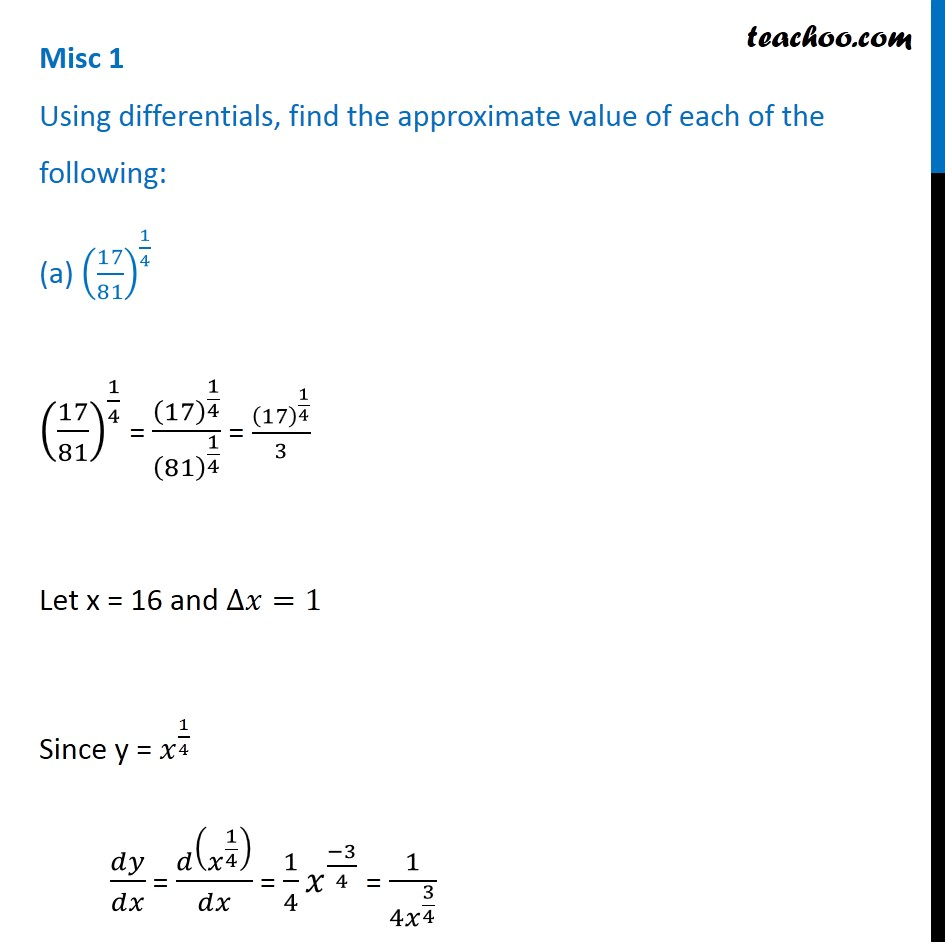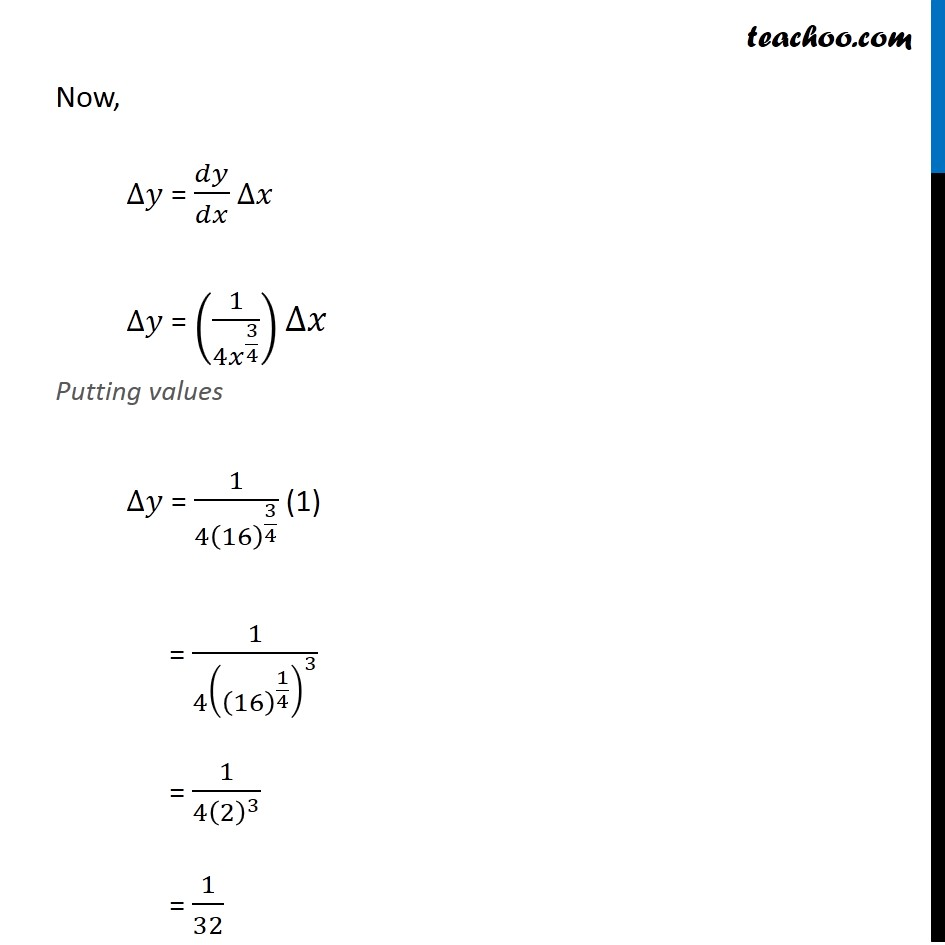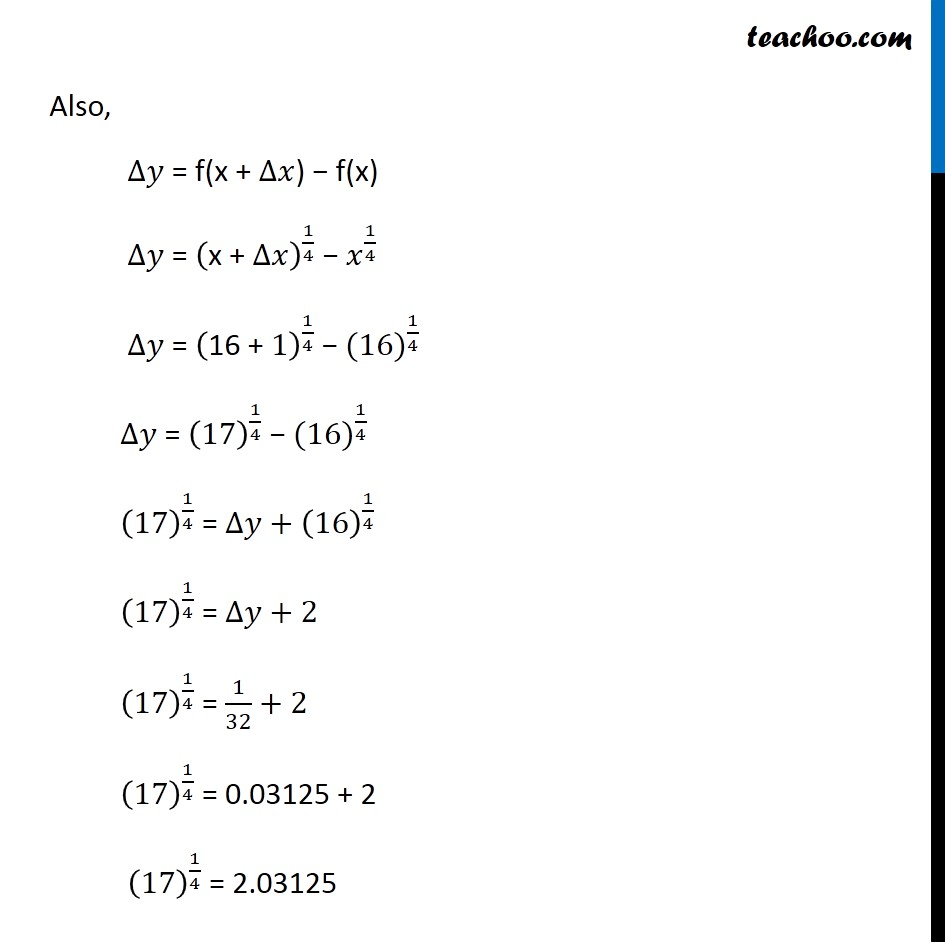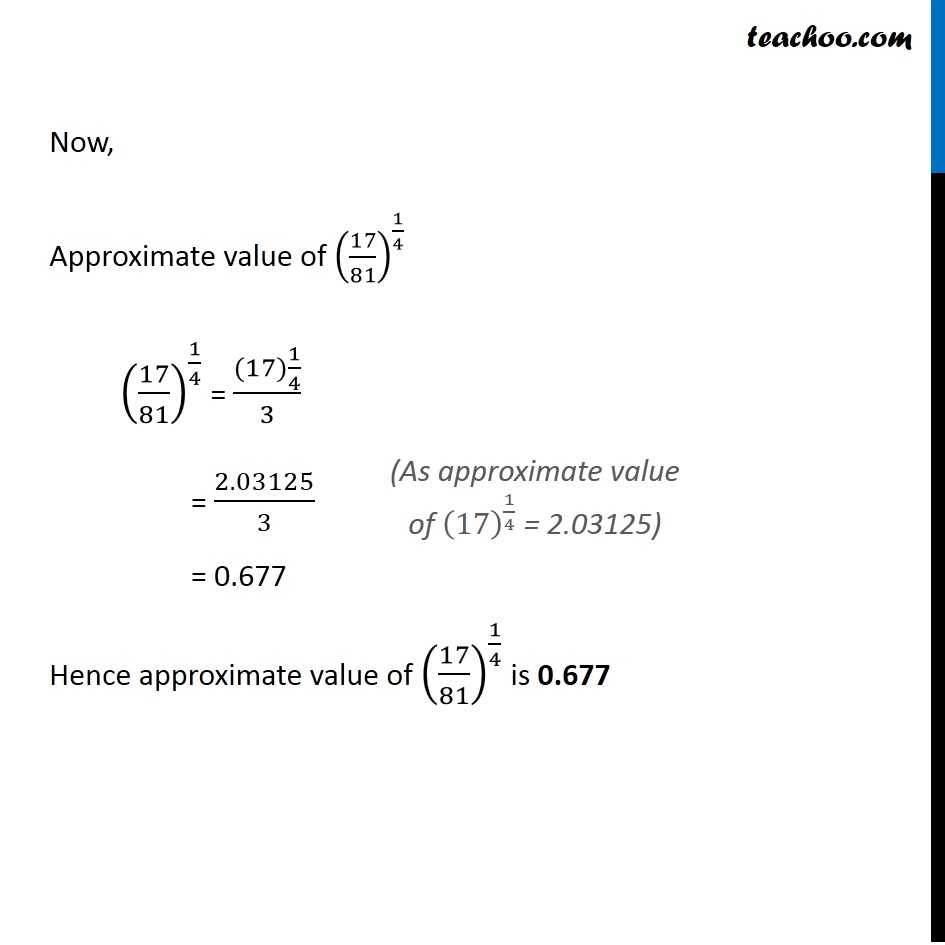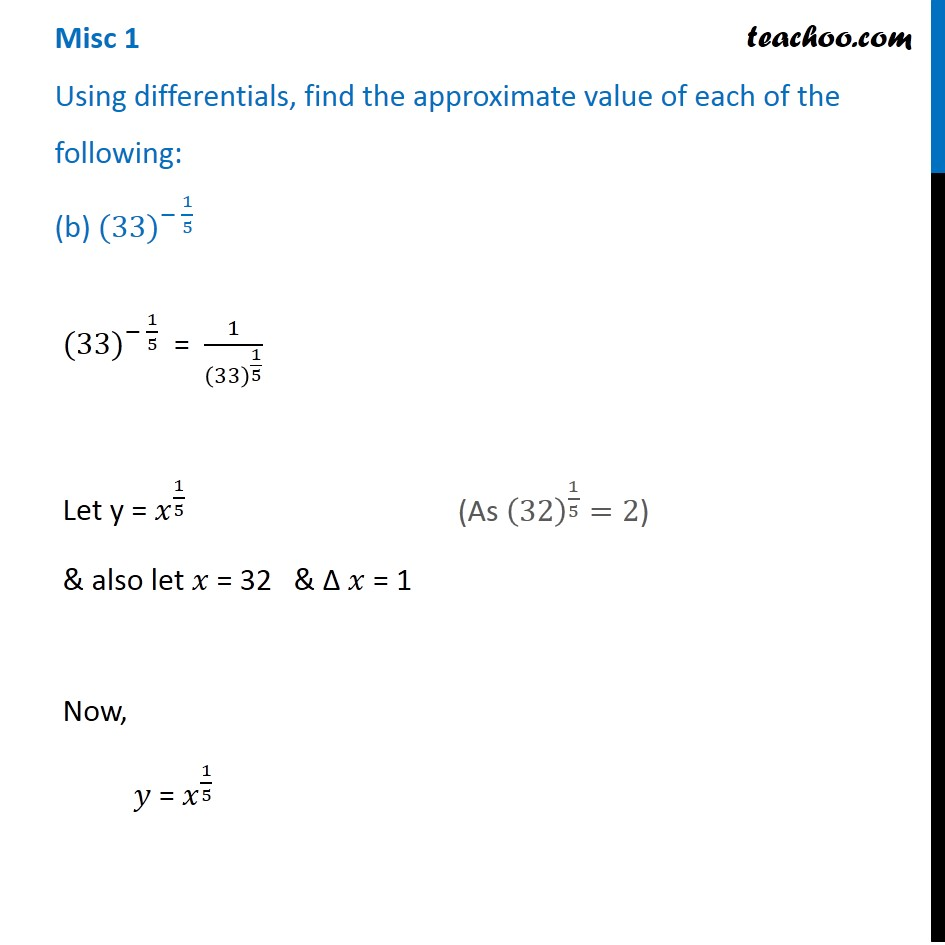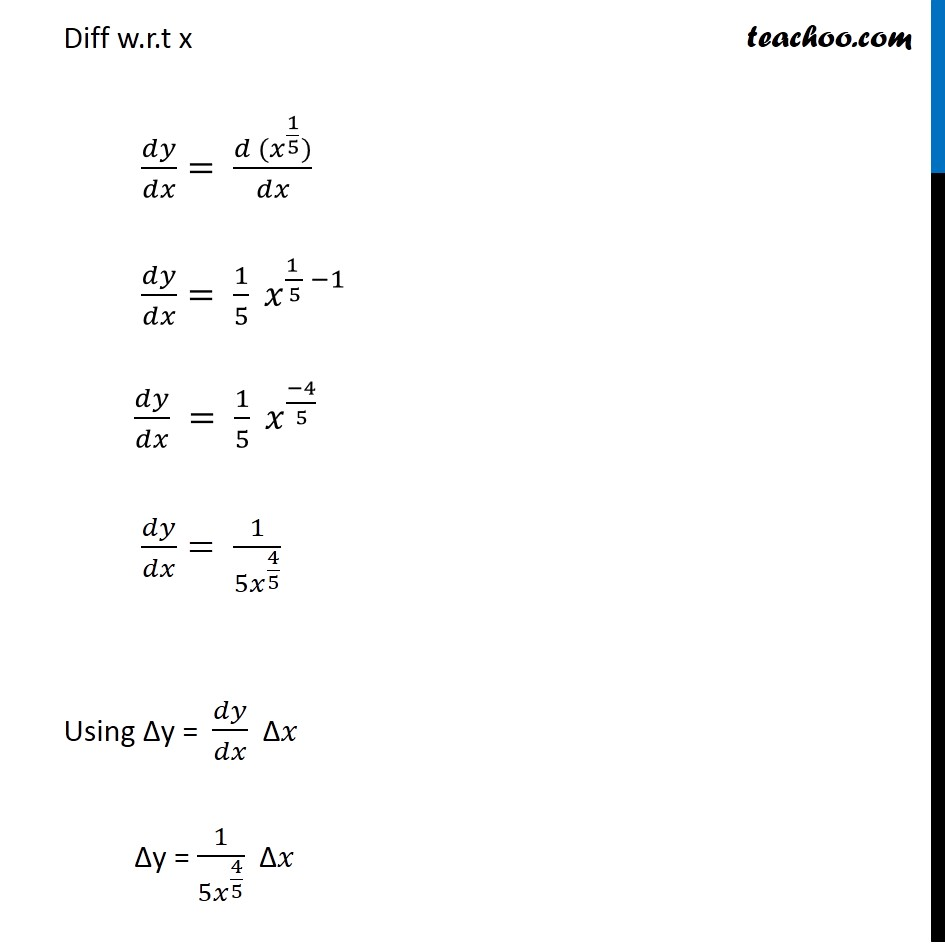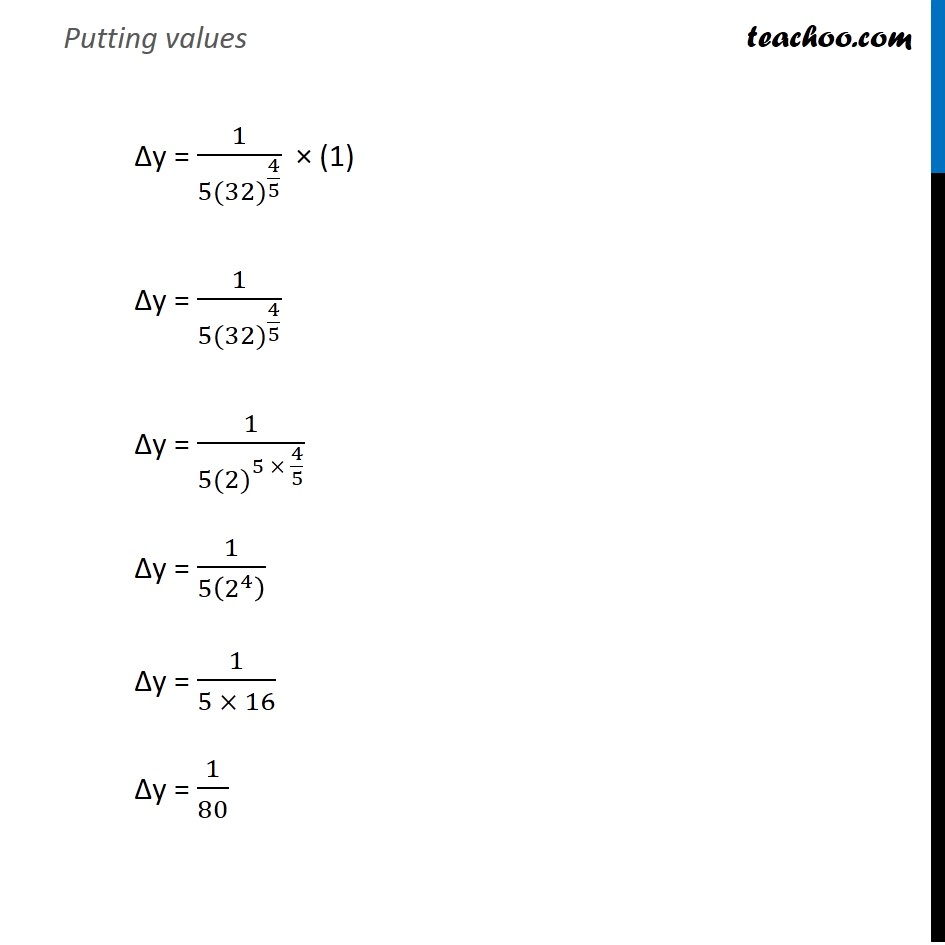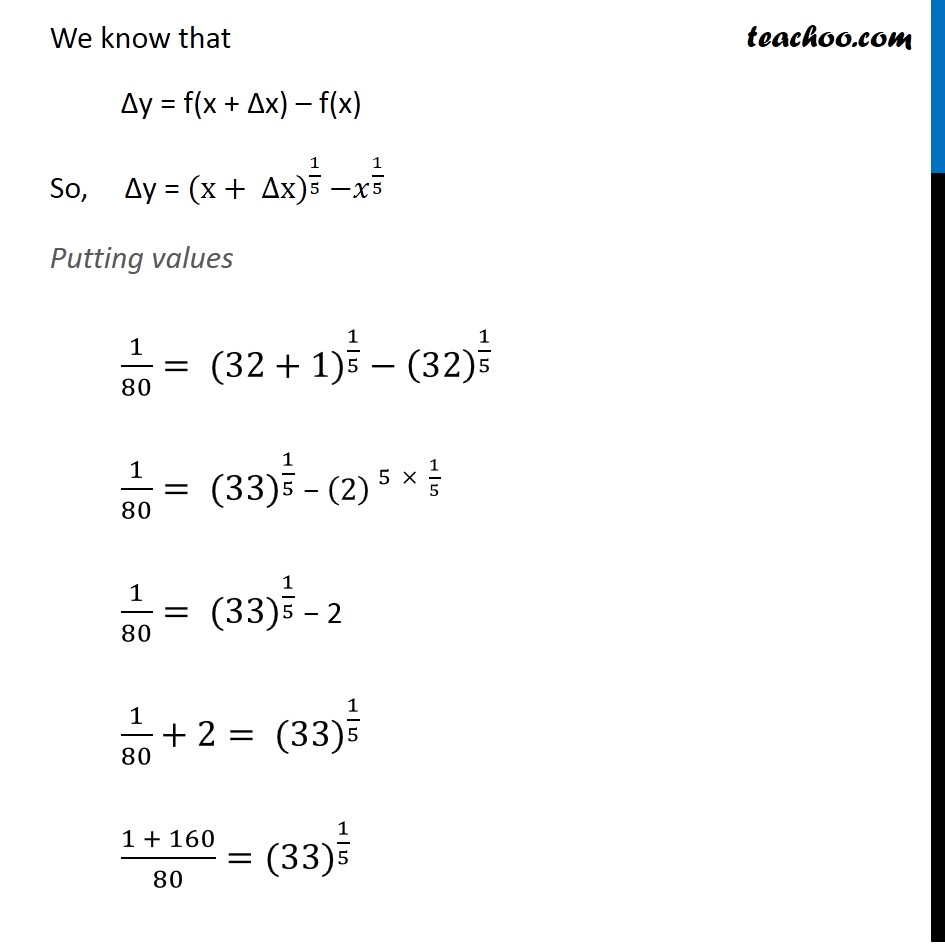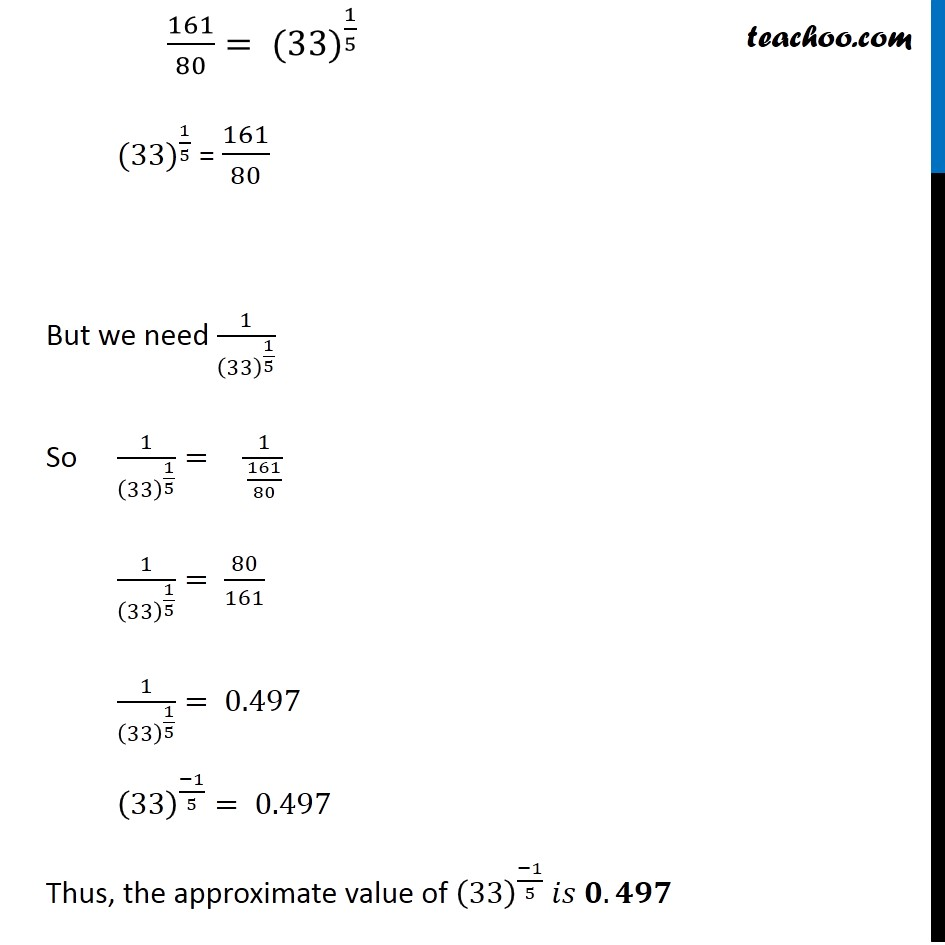1. Chapter 6 Class 12 Application of Derivatives
2. Serial order wise
3. Miscellaneous

Transcript

Misc 1 Using differentials, find the approximate value of each of the following: (a) (17/81)^(1/4) (17/81)^(1/4) = (17)^(1/4)/(81)^(1/4) = (17)^(1/4)/3 Let x = 16 and ∆𝑥=1 Since y = 𝑥^(1/4) 𝑑𝑦/𝑑𝑥 = 𝑑(𝑥^(1/4) )/𝑑𝑥 = 1/4 𝑥^((−3)/4) = 1/(4𝑥^(3/4) ) Now, ∆𝑦 = 𝑑𝑦/𝑑𝑥 ∆𝑥 ∆𝑦 = (1/(4𝑥^(3/4) ))∆𝑥 Putting values ∆𝑦 = 1/(4(16)^(3/4) ) (1) = 1/(4((16)^(1/4) )^3 ) = 1/(4(2)^3 ) = 1/32 Also, ∆𝑦 = f(x + ∆𝑥) − f(x) ∆𝑦 = ("x + " ∆𝑥)^(1/4) − 𝑥^(1/4) ∆𝑦 = ("16 + " 1)^(1/4) − 〖(16)〗^(1/4) ∆𝑦 = (17)^(1/4) − 〖(16)〗^(1/4) (17)^(1/4) = ∆𝑦+(16)^(1/4) (17)^(1/4) = ∆𝑦+2 (17)^(1/4) = 1/32+2 (17)^(1/4) = 0.03125 + 2 (17)^(1/4) = 2.03125 Now, Approximate value of (17/81)^(1/4) (17/81)^(1/4) = ((17)1/4)/3 = 2.03125/3 = 0.677 Hence approximate value of (17/81)^(1/4) is 0.677 (As approximate value of (17)^(1/4) = 2.03125) Misc 1 Using differentials, find the approximate value of each of the following: (b) 〖(33)〗^(− 1/5) 〖(33)〗^(− 1/5) = 1/(33)^(1/5) Let y = 𝑥^(1/5) & also let 𝑥 = 32 & ∆ 𝑥 = 1 Now, 𝑦 = 𝑥^(1/5) (As (32)^(1/5)=2) Diff w.r.t x 𝑑𝑦/𝑑𝑥= (𝑑 (𝑥^(1/5)))/𝑑𝑥 𝑑𝑦/𝑑𝑥= 1/5 𝑥^((1 )/5 −1) 𝑑𝑦/𝑑𝑥 = 1/5 𝑥^((−4)/5) 𝑑𝑦/𝑑𝑥= 1/〖5𝑥〗^(4/5) Using ∆y = 𝑑𝑦/𝑑𝑥 ∆𝑥 ∆y = 1/〖5𝑥〗^(4/5) ∆𝑥 Putting values ∆y = 1/〖5(32)〗^(4/5) × (1) ∆y = 1/〖5(32)〗^(4/5) ∆y = 1/〖5(2)〗^(5 × 4/5) ∆y = 1/5(2^4 ) ∆y = 1/(5 × 16) ∆y = 1/80 We know that ∆y = f(x + ∆x) – f(x) So, ∆y = 〖(x+ Δx)〗^(1/5) 〖−𝑥〗^(1/5) Putting values 1/80= 〖(32+1)〗^(1/5) − (32)^(1/5) 1/80= 〖(33)〗^(1/5) − 〖(2) 〗^(5 × 1/5) 1/80= 〖(33)〗^(1/5) − 2 1/80+2= 〖(33)〗^(1/5) (1 + 160)/80=〖(33)〗^(1/5) 161/80= 〖(33)〗^(1/5) 〖(33)〗^(1/5) = 161/80 But we need 1/(33)^(1/5) So 1/(33)^(1/5) = 1/(161/80) 1/(33)^(1/5) = 80/161 1/(33)^(1/5) = 0.497 (33)^((−1)/5)= 0.497 Thus, the approximate value of (33)^((−1)/5) 𝑖𝑠 𝟎.𝟒𝟗𝟕

Miscellaneous